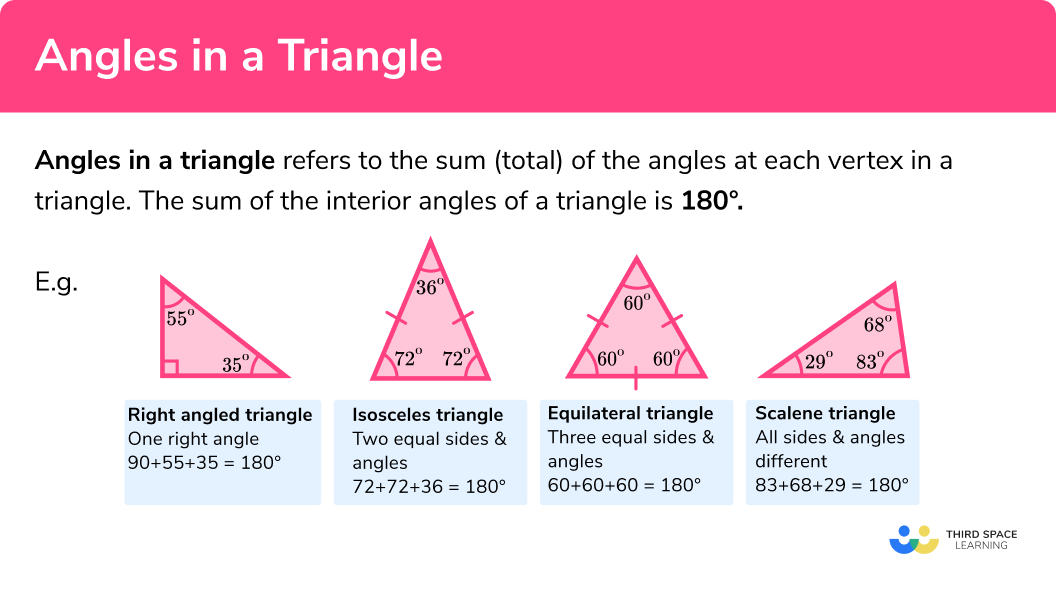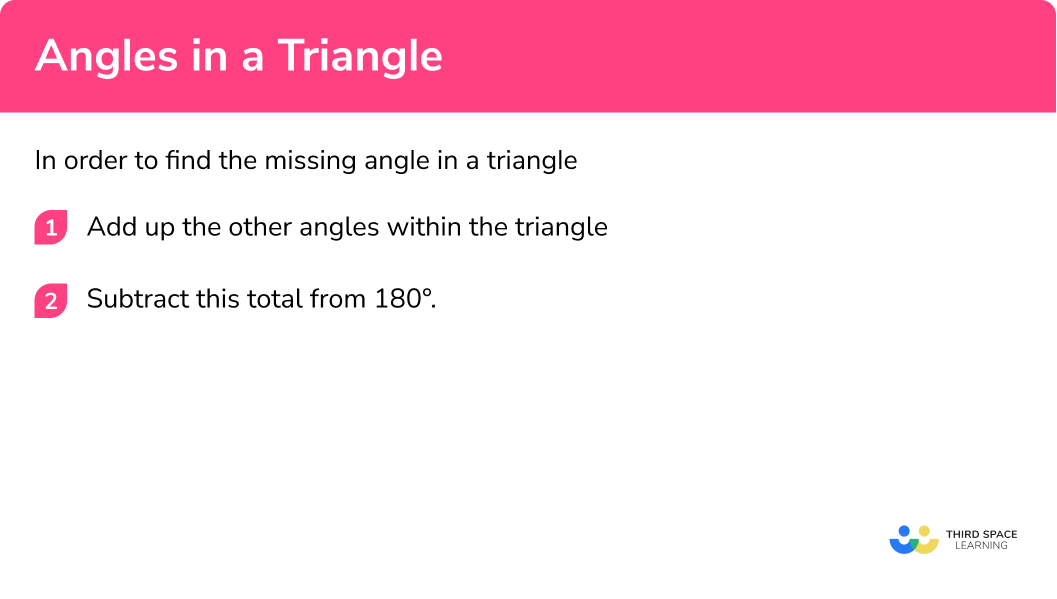# Angles In A Triangle

Here is everything you need to know about angles in a triangle including what the angles in a triangle add up to, how to find missing angles, and how to use this alongside other angle facts to form and solve equations.

There are also angles in a triangle worksheets based on Edexcel, AQA and OCR exam questions, along with further guidance on where to go next if you’re still stuck.

## What are angles in a triangle?

All triangles have interior angles that add up to 180º .

Angles in a triangle are the sum (total) of the angles at each vertex in a triangle.

We can use this fact to calculate missing angles by finding the total of the given angles and subtracting it from 180º .

This is true for all types of triangles.

• Right Angle Triangle: One 90° angle, the other two angles will have a total of 90°.
• Isosceles Triangle: Two equal sides and angles.
• Equilateral Triangle: All three angles are 60°.
• Scalene Triangle: All three angles are different.

Examples:

### What are angles in a triangle?## How to find a missing angle in a triangle

In order to find the missing angle in a triangle:

1. Add up the other angles within the triangle.
2. Subtract this total from 180º .

### Explain how to find a missing angle in a triangle in 2 steps### Related lessons on angles in polygons

Angles in a triangle is part of our series of lessons to support revision on angles in polygons. You may find it helpful to start with the main angles in polygons lesson for a summary of what to expect, or use the step by step guides below for further detail on individual topics. Other lessons in this series include:

## Finding missing angles examples

### Example 1: scalene triangle

Work out the size of the angle labelled a in the following triangle.

1. We are given the angles 57º and 79º . Add these together.

$57 +79 = 136^{\circ}$

2Subtract 136º from 180º .

$180 – 136 = 44^{\circ}$

$a = 44^{\circ}$

### Example 2: right angled triangle

Find the angle labelled b in the following triangle.

$90 + 19 = 109^{\circ}$

$180-109=71^{\circ}$

$b = 71^{\circ}$

### Example 3: isosceles triangle

Find the angle labelled c in the following triangle.

When two sides of a triangle are equal, the angles at the ends of those sides will also be equal.

$64+64=128^{\circ}$

$180-128=52^{\circ}$

$c = 52^{\circ}$

## How to find one of the two equal angles in an isosceles triangle

1. Subtract the given angle from 180º .
2. Divide by 2 .

### Example 4: equal angles in an isosceles triangle

Find the size of the angle labelled d in the triangle below.

$180-110=70^{\circ}$

The other two angles in this triangle add up to 70º .

$70 \div 2=35^{\circ}$

$d = 35^{\circ}$

## How to use angle facts to solve problems

Sometimes the problem will involve using other angle facts.
Let’s recap some of the other angle facts we know:

1. Use angle facts to fill in any possible angles.
2. Use these angles to calculate missing angles in the triangle.

These steps are interchangeable and may need to be repeated for more difficult problems.

### Example 5: using angles at a point

Find the size of the angle labelled e .

$360-310=50^{\circ}$

$100+50=150$

$180-150=30^{\circ}$

$e = 30^{\circ}$

### Example 6: using opposite angles

$90+61=151$

$180-151=29^{\circ}$

$f = 29^{\circ}$

### Example 7: two different triangles

Find the size of the angle labelled g .

$48+18=66$

$180-66=114^{\circ}$

$180-114=66^{\circ}$

$66+66=132$

$180-132=48^{\circ}$

$g = 48^{\circ}$

## How to work out angles in a triangle with algebra

We can use the fact that the angles in a triangle add up to 180º to form equations which we can then solve to find the values of the angles in the triangle.

1.  Add together the expressions for each angle and simplify.
2.  Put the simplified expression equal to 180º .
3. Solve the equation.
4.  Substitute your value back in to find the angles in the triangle.

### Example 8: angles involving algebra

Find the size of each angle in this triangle.

$4h + 3h + 2h = 9h$

$9h = 180$

$h = 20$

\begin{array}{l} 2 \times 20 = 40\\\\ 3 \times 20 = 60\\\\ 4 \times 20 = 80 \end{array}

The three angles are 40º , 60º and 80º .

### Example 9: angles involving algebra

Find the size of each angle in this right-angled triangle.

$3i + 25 + 2i – 5 + 90 = 5i + 110$

$5i + 110 = 180$

$5i = 70$

$i = 14$

\begin{array}{l} 3 \times 14+25=67\\\\ 2 \times 14-5=23 \end{array}

The three angles are 23º , 67º and 90º .

### Common misconceptions

• Incorrect angle sum

Using 360º instead of 180º for the sum of the angles of the triangle.

• Equal angles in an isosceles triangle

Selecting the wrong angles when identifying the equal angles in an isosceles triangle (particularly a problem when the equal angles are not at the bottom). The angle that is different in an isosceles triangle is the one between the two sides with equal length.

### Practice angles in a triangle questions

1. Find the angle labelled z in the following triangle.33^{\circ}123^{\circ}57^{\circ}213^{\circ}90+57=147

180-147=33^{\circ}

2. Find the angle labelled y .51^{\circ}258^{\circ}78^{\circ}39^{\circ}This is an isosceles triangle and the two angles at the bottom of the triangle are equal.

51+51=102

180-102=78^{\circ}

3. Find the angle x in the following triangle.42^{\circ}69^{\circ}138^{\circ}48^{\circ}This is an isosceles triangle and the two angles on the right are equal.

180-42=138

138 \div 2 = 69^{\circ}

4. What is the size of each angle in an equilateral triangle?

60^{\circ}90^{\circ}30^{\circ}180^{\circ}All three angles in an equilateral triangle are equal so

180 \div 3 = 60^{\circ}

5. Find the size of the angle labelled w in the following triangle.24^{\circ}156^{\circ}48^{\circ}78^{\circ}The angle opposite 24^{\circ} is also 24^{\circ} since vertically opposite angles are equal.

The triangle is an isosceles triangle and the two angles on the left are the same size.

180-24=156

156 \div 2 = 78^{\circ}

6.  Find the angle labelled v .51^{\circ}20^{\circ}129^{\circ}31^{\circ}Looking at the left hand triangle frist, we can find the missing angle in that triangle:

90+39=129

180-129=51^{\circ}

We can then use the fact that angles on a straight line add up to 180^{\circ} to find the unlabelled angle in the right hand triangle:

180-51=129^{\circ}We can then find angle v :

129+31=160^{\circ}

180-160=20^{\circ}

7. Write an equation involving u and use it to find the size of each angle in the following triangle.176^{\circ}, 112^{\circ}, 76^{\circ}102.5^{\circ}, 72.5^{\circ}, 46.25^{\circ}86^{\circ}, 56^{\circ}, 38^{\circ}78^{\circ}, 48^{\circ}, 34^{\circ}2u+20+2u-10+u+5=5u+15

Therefore

\begin{aligned} 5u+15&=180\\\\ 5u&=165\\\\ u&=33^{\circ} \end{aligned}

2 × 33+20=86^{\circ}

2 × 33-10=56^{\circ}

33+5=38^{\circ}

### Angles in a triangle GCSE questions

1. Find the size of angle x given that the exterior angle shown is 153^{\circ} .(2 marks)

180-153=27^{\circ}

(1)

90 + 27 = 177

180-117=63^{\circ}

(1)

2. (a) Calculate the size of angle ACE .

(b) Show that BCD is an isosceles triangle.(5 marks)

(a)

90 + 36 = 126

(1)

180-126=54^{\circ}

(1)

(b)

Angle CBD :

= 180 – 117

=63^{\circ}

Angle BDC

63 + 54 = 117

180-117=63^{\circ}

(1)

Two angles equal therefore isosceles

(1)

3. Work out the size of the smallest angle in the right angled triangle.(4 marks)

3x – 10 + 2x + 55 + 90 (= 5x + 135)

(1)

5x + 135 = 180

(1)

x = 9

(1)

3\times 9-10=17^{\circ}

(1)

## Learning checklist:

You have now learned how to:

• Use the sum of the angles of a triangle to find missing angles
• Apply other angle facts to find missing angles in triangle problems
• Form and solve equations using the sum of the angles in a triangle

## Still stuck?

Prepare your KS4 students for maths GCSEs success with Third Space Learning. Weekly online one to one GCSE maths revision lessons delivered by expert maths tutors.

Find out more about our GCSE maths tuition programme.